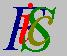Mauro Murzi's pages on Philosophy of Science - Logical Positivism
The Language of Science

# [2. The Main Philosophical Tenets of Logical Positivism.]

## c. The Language of Science.

According to logical positivism, a scientific theory is an axiomatic system that acquires an empirical interpretation only by means of appropriate statements called rules of correspondence, which establish a correlation between real objects (or real processes) and the abstract concepts of the theory. Without such type of statements a theory lacks of a physical interpretation and it is not verifiable, but it is an abstract formal system, whose only requirement is axioms consistency.

The language of a theory includes three kinds of terms:

1. Logical terms, which include all mathematical terms.
2. Observational terms, which denote objects or properties that can be directly observed or measured.
3. Theoretical terms, which denote objects or properties we cannot observe or measure but we can only infer from direct observations.

According to this distinction, the statements of a theory are divided in three sets:

1. Logical statements, which include only logical terms.
2. Observational statements, which include observational and logical terms.
3. Theoretical statements, which include theoretical, observational and logical terms. Theoretical statements are divided in:
• Pure theoretical statements, which do not include observational terms.
• Mixed theoretical statements, which include observational terms. Rules of correspondence belongs to this set of statements.

The following table represents the diverse kinds of statements.

 Statements L-statements O-statements T-statements Pure T-statements Mixed T-statements L-terms L-terms O-terms L-terms T-terms L-terms O-terms T-terms
(Abbreviations: L=Logical, O=Observational, T=Theoretical)

With respect to the method of ascertaining their truth or falsity, the statements of a scientific theory are divided in two sets:

1. Analytic a priori statements, whose truth is based on the meaning of the terms of the language. They include logical statements, whose truth is based only on the rules of logic and mathematics.
2. Synthetic a posteriori statements, which are the not-analytic statements.

Another distinction is between:

1. Ax-true or Ax-false statements, which are either a logical consequence of the axioms of the theory or incompatible with the axioms.
2. Contingent statements, which are independent from the axioms of the theory.

The following table represents the diverse kinds of statements.

 True False Ax-true Contingent Ax-false Analytic a priori true Synthetic a posteriori Analytic a priori false Logical true Possible Logical false

The main points of this thesis about the structure of scientific theories are:

• The distinction between observational and theoretical terms
• The distinction between synthetic and analytic statements
• The distinction between theoretical axioms and rules of correspondence
• The deductive nature of scientific theories.

These four points are linked together. Rules of correspondence give an empirical meaning to theoretical terms and are analytic, while theoretical axioms express the empirical portion of the theory and are synthetic. A theory must be a deductive system; otherwise, a formal distinction between the various kinds of sentences and terms is impossible.